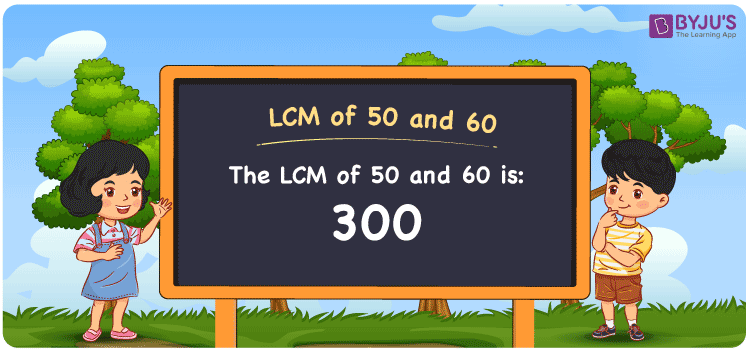Checkout JEE MAINS 2022 Question Paper Analysis : Checkout JEE MAINS 2022 Question Paper Analysis :

# LCM of 50 and 60

LCM of 50 and 60 is 300. In Maths, the value which is divisible by the two numbers indicates the LCM. Least common multiple of 50 and 60 is the smallest number which can be obtained from the common multiples. (50, 100, 150, 200, 250, 300, ….) and (60, 120, 180, 240, 300,….) are the first few multiples of 50 and 60. The LCM can be calculated using various methods like prime factorisation, division and by listing the multiples.

## What is LCM of 50 and 60?

The answer to this question is 300. The LCM of 50 and 60 using various methods is shown in this article for your reference. The LCM of two non-zero integers, 50 and 60, is the smallest positive integer 300 which is divisible by both 50 and 60 with no remainder.## How to Find LCM of 50 and 60?

LCM of 50 and 60 can be found using three methods:

• Prime Factorisation
• Division method
• Listing the multiples

### LCM of 50 and 60 Using Prime Factorisation Method

The prime factorisation of 50 and 60, respectively, is given by:

50 = 2 x 5 x 5 = 2¹ x 5²

60 = 2 x 2 x 3 x 5 = 2² x 3¹ x 5¹

LCM (50, 60) = 300

### LCM of 50 and 60 Using Division Method

We’ll divide the numbers (50, 60) by their prime factors to get the LCM of 50 and 60 using the division method (preferably common). The LCM of 50 and 60 is calculated by multiplying these divisors.

 2 50 60 2 25 30 3 25 15 5 25 5 5 5 1 x 1 1

No further division can be done.

Hence, LCM (50, 60) = 300

### LCM of 50 and 60 Using Listing the Multiples

To calculate the LCM of 50 and 60 by listing out the common multiples, list the multiples as shown below:

 Multiples of 50 Multiples of 60 50 60 100 120 150 180 200 240 250 300 300 360

The smallest common multiple of 50 and 60 is 300.

Therefore LCM (50, 60) = 300

## Video Lesson on Applications of LCM## LCM of 50 and 60 Solved Examples

Find the LCM if the product of two numbers is 3000 and the GCD is 10.

Solution:

It is given that

Product of two numbers = 3000

GCD = 10

We know that,

LCM x GCD = Product of two numbers

LCM = Product/GCD

LCM = 3000/10

LCM = 300

Hence, the LCM is 300.

## Frequently Asked Questions on LCM of 50 and 60

### What is the LCM of 50 and 60?

The LCM of 50 and 60 is 300. To find the LCM, we should first know the multiples of 50 and 60 and the smallest multiple which is divisible by 24 and 36 has to be determined.

### What are the methods used to find the LCM of 50 and 60?

The methods used to find the LCM of 50 and 60 are Prime Factorization Method, Division Method and Listing multiples.

### Calculate the GCF if the LCM of 50 and 60 is 300.

LCM x GCF = 50 x 60

Given

LCM of 50 and 60 = 300

300 x GCF = 3000

GCF = 3000/300 = 10